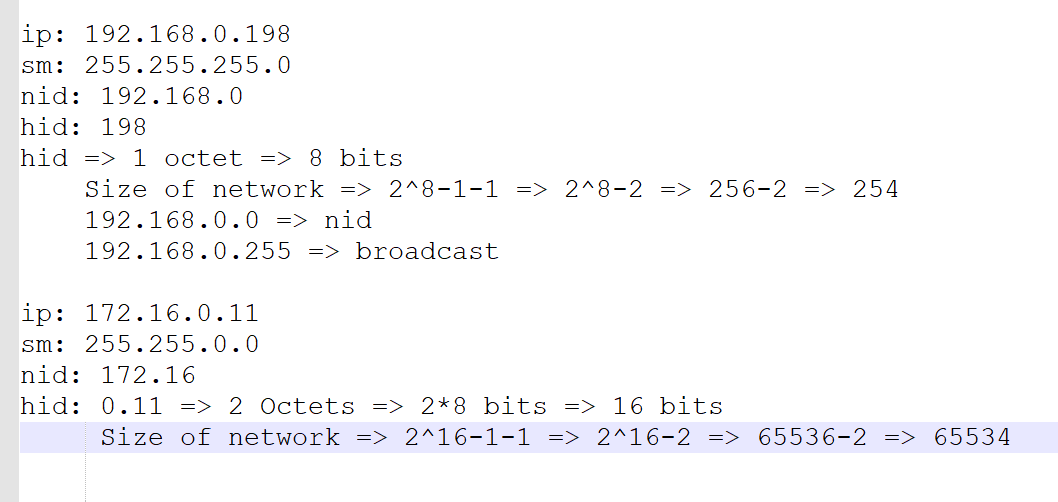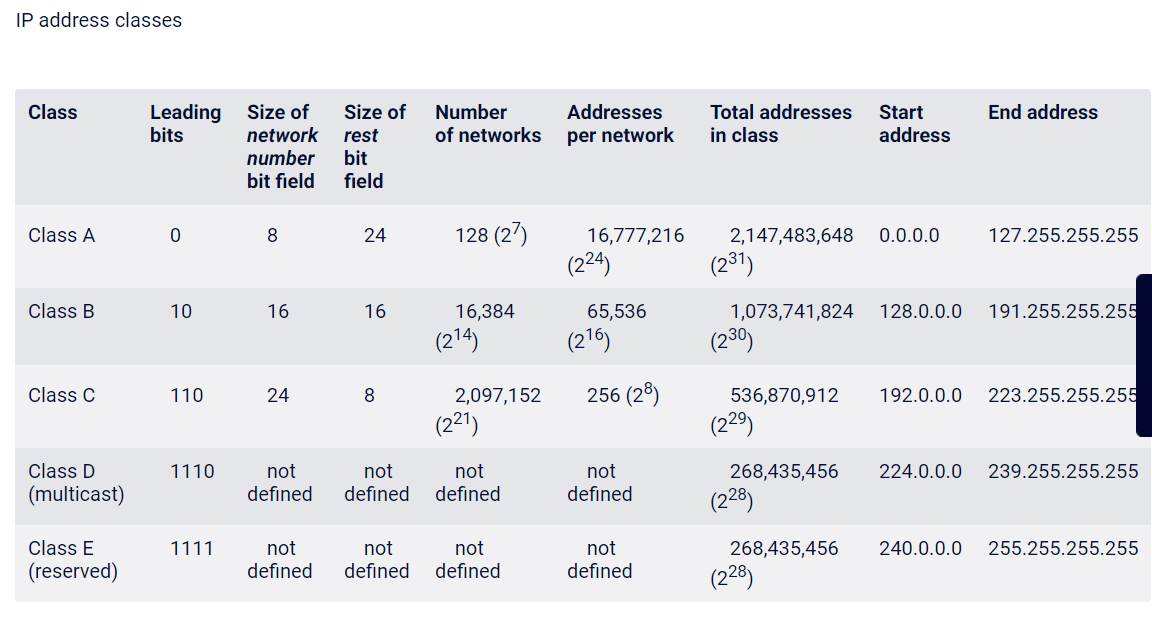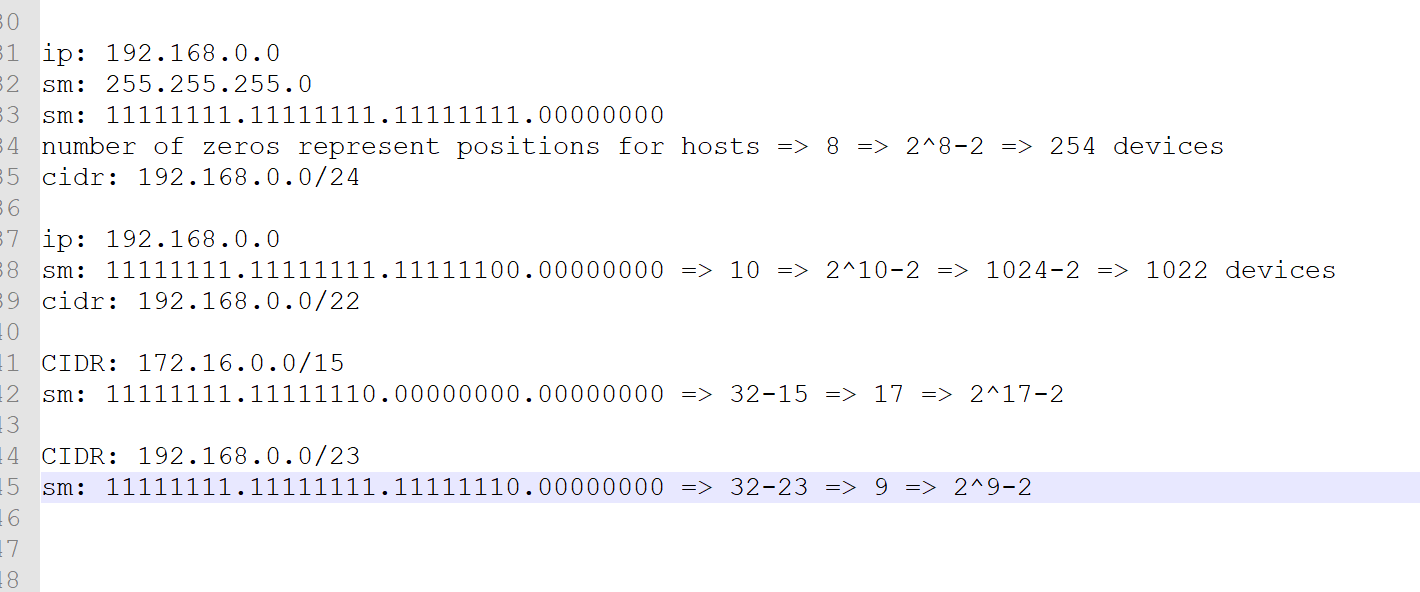### Networking Basics

• IP Address is a unique id given to a device in a network

• IP Address enables data communication b/w hosts

• We have two IP Addressing formats

• IPv4
• IPv6
• IPv4 Addresses:

• consists of 32 bits divided into 4 sections called as octects
• Each octet contains 8 bits (1 byte) separate by a dot
• Sample IPv4 Addresses
``````192.168.0.198
``````• IP Address is combination of

• network id
• host id
• It is not possible to tell what is network id or what is host id just by ip address, we need a subnet mask for that.

• Example of using sm to calculate size of the network• if we continue with subnet mask as decimal number we have 3 possible sizes for host id

• one octet => 2^8-2 => 254 devices
• two octets => 2^16-2 => 65534 devices
• three octets => 2^24-2 => 16777214 devices
• Lets assume you are trying to create a network with 500 devices

• To assign an IP Address range to your network there are two popular addressing schemes

• Classful Addressing
• Classless Addressing
• Classful Addressing:

• This was introduced in 1981 where IPv4 Addresses were divided into 5 classes (A to E)• Classless Interdomain routing (CIDR) was introduced in 1993 to replace classfull addressing. It uses Variable length subnet masks

#### CIDR

• In classfull addressing we have looked at subnet mask as a decimal number so we had 3 possibilities
• 255.255.255.0 => one octet (n/w size)
• 255.255.0.0 => two octets (n/w size)
• 255.0.0.0 => three octets (n/w size)
• In this approach we look at subnet mask as a binary numberThis site uses Akismet to reduce spam. Learn how your comment data is processed.